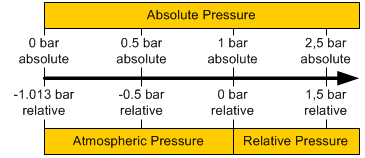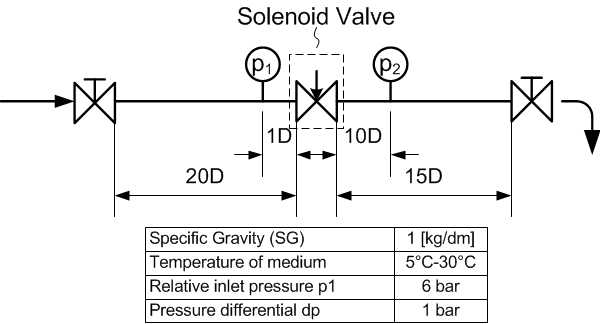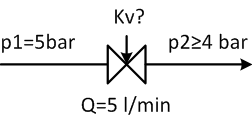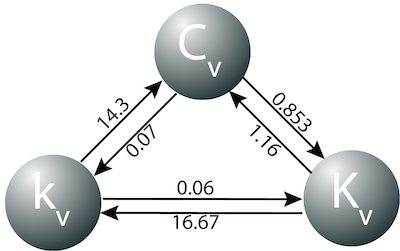# Flow Principles for Valves

## Pressure

Pressure is defined as the amount of force acting per unit area:

p=F/A

where:

• p = pressure in Pascal (N/m2)
• F = force in Newton (N)
• A = surface (m2)

Pascal is a small unit, therefore bar is often used instead: 1 bar = 105 Pa. When making calculations, be aware on the difference between absolute and relative pressure. On average, the atmospheric pressure is 1.013 bar. The diagram below clearly shows the relation between absolute and relative pressure.## Flow coefficient Kv

Definition Kv-value: Amount of flow (m3/hour) of water of 20°C in a valve with a pressure loss of 1 bar.

The amount of flow of a fluid through a (solenoid) valve can easily be calculated with flow coefficient Kv. Please note that for gases (e.g. air) a different formula is used (see correction factor gases). The Kv-value expresses the flow rate of water in m3/hour in a valve with a pressure loss of 1 bar and a temperature of 20°C. Pay attention that the Kv-value is expressed in m3/hour, while the kv-value (lowercase!) is expressed in l/min. To calculate the flow rate the following formula is used:where:

• Q = flow rate of liquid (m3/hour)
• Kv = flow coefficient (m3/hour)
• SG = Specific Gravity (=1 for water)
• dp = pressure differential over the valve (bar)

The Kv-value of a valve is determined by a standardised test according to VDI/VDE 2173. For this purpose a test set-up is used, such as shown in the following schematic drawing:### Example

Consider a circuit with a solenoid valve with an inlet pressure (water) of 5 bars. The required flow rate is at least 5 litres per minute. The maximum allowed pressure loss is 1 bar, therefore the outlet pressure is minimally 4 bars. What is the minimum required Kv-value?5 l/min = 0.3 m3/hourThe Kv-value must be at least 0.3. In the shop you can use a filter in the left column to select valves with your required Kv-value.

## Correction factor for gases

When using gases, a different formula for the flow rate should be used. Also, a distinction between subsonic and supersonic flows is made. "Qn" indicates that the formula is valid for standard conditions (20°C). The flow rate is expressed in Nm3/h (Normal cubic metre per hour).

### Subsonic### SupersonicWhere:

• dP = pressure loss (bar)
• Kv = flow coefficient (m3/hour)
• p1 and p2 are respectively the absolute inlet and outlet pressure (bar)
• ? = specific gravity at standard conditions (20°C) in kg/m3
• T = absolute temperature in Kelvin (0K = -273.15°C)

## Cv-value

Sometimes, the Cv value is given instead of the Kv value. The Cv value can be converted to the Kv-value with a conversion factor:

• Cv = 1.16 Kv
• Kv = 0.853 Cv

The diagram below is a nice tool to simplify the conversion between the different coefficients. The conversion factor between two coefficients is indicated with arrows.Kv (with a capital K) expresses the flow rate in m3/hour, kv (lowercase) in l/min.

## Valves in parallel

If several valves are connected in parallel, the Kv value can be determined simply by adding up all Kv values.

### Tameson's monthly newsletter

• Who is it for: You! Existing customers, new customers, and anyone seeking fluid control information.
• Why Tameson's monthly newsletter: It is straight forward, no nonsense, and full of relevant information about the fluid control industry once a month.
• What's in it: New product announcements, technical articles, videos, special pricing, industry information, & much more that you'll have to subscribe to see!